# Integrals in involution

(diff) ← Older revision | Latest revision (diff) | Newer revision → (diff)

Solutions of differential equations whose Jacobi brackets vanish identically. A function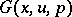ofvariables,,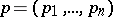is a first integral of the first-order partial differential equation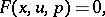(1)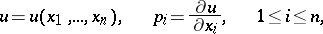if it is constant along each characteristic of this equation. Two first integrals,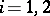, are in involution if their Jacobi brackets vanish identically in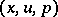: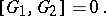(2)

More generally, two functionsare in involution if condition (2) holds. Any first integralof equation (1) is in involution with; the last function itself is a first integral.

These definitions can be extended to a system of equations(3)

Here the first integral of this systemcan be regarded as a solution of the system of linear equations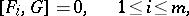(4)

with unknown function.

If (3) is an involutional system, then (4) is a complete system. It is in involution if the functions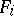in (3) do not depend on.

How to Cite This Entry:
Integrals in involution. Encyclopedia of Mathematics. URL: http://encyclopediaofmath.org/index.php?title=Integrals_in_involution&oldid=15040
This article was adapted from an original article by A.P. Soldatov (originator), which appeared in Encyclopedia of Mathematics - ISBN 1402006098. See original article